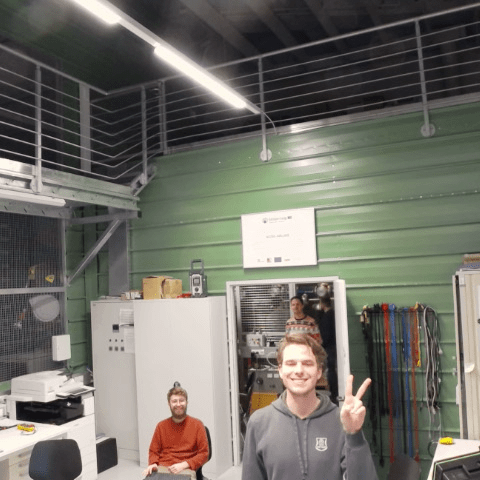# SIFT Keypoint Matching using Python OpenCV

I have been working on SIFT based keypoint tracking algorithm and something happened on Reddit. Kat wanted this is Python so I added this feature in SimpleCV. Here’s the pull request which got merged.

## SIFT KeyPoints Matching using OpenCV-Python:

To match keypoints, first we need to find keypoints in the image and template. OpenCV Python version 2.4 only has SURF which can be directly used, for every other detectors and descriptors, new functions are used, i.e. FeatureDetector_create() which creates a detector and DescriptorExtractor_create() which creates a descriptor to extract keypoints.

Import required modules

```import cv2
import numpy as np
import itertools
```

```img = cv2.imread("kpimg.png")
```

#### Find Keypoints:

```detector = cv2.FeatureDetector_create("SIFT")
descriptor = cv2.DescriptorExtractor_create("SIFT")

skp = detector.detect(img)
skp, sd = descriptor.compute(img, skp)

tkp = detector.detect(template)
tkp, td = descriptor.compute(template, tkp)
```

skp is a list of all the keypoints found on the image. sd is the descriptor for the image.
tkp is a list of all the keypoints found on the template. td is the descriptor for the template.

Properties of cv2.KeyPoint:

• pt – coordinates of the keypoint
• size – diameter of the meaningful keypoint neighborhood
• angle – computed orientation of the keypoint. range [0,360) degrees.
• response – the response by which the most strong keypoints have been selected. Can be used for further sorting or subsampling
• octave – octave (pyramid layer) from which the keypoint has been extracted

Matching Keypoints using FLANN:
It was very easy to match keypoints in C++ using FlannMatcher, but it’s a bit difficult to that in Python.

```flann_params = dict(algorithm=1, trees=4)
flann = cv2.flann_Index(sd, flann_params)
idx, dist = flann.knnSearch(td, 1, params={})
del flann
```

This method does a fast local approximate nearest neighbors (FLANN) calculation between two sets of feature vectors. The result are two numpy arrays the first one is a list of indexes of the matches and the second one is the match distance value. For the match indices or idx, the index values correspond to the values of td, and the value in the array is the index in td.
i.e. j = idx[i] is where td[i] matches sd[j].
The second numpy array, at the index i is the match distance between td[i] and sd[j]. Lower distances mean better matches.

Perform the similar exercise on template to match keypoints of template to the image so that any erroneous keypoint match may be eliminated.

Sorting keypoint matches according to the distance:

```dist = dist[:,0]/2500.0
dist = dist.reshape(-1,).tolist()
idx = idx.reshape(-1).tolist()
indices = range(len(dist))
indices.sort(key=lambda i: dist[i])
dist = [dist[i] for i in indices]
idx = [idx[i] for i in indices]
```

After sorting the keypoints according to the distance, filter keypoints by setting a cut-off limit of distance.

```skp_final = []
for i, dis in itertools.izip(idx, dist):
if dis < distance:
skp_final.append(skp[i])
else:
break
```

Since keypoints are already sorted according to distance, once the cut-off is surpassed, it is redundant to check for other keypoints.

Do this for both template and image keypoints matches.

Draw Keypoints:
Now, we have matched keypoints. We need to draw them on a new image which consist of the image and the template. First, I thought it would be too difficult to put two images side by side but I underestimated numpy arrays. Things got real easy.

```h1, w1 = img.shape[:2]
h2, w2 = template.shape[:2]
nWidth = w1+w2
nHeight = max(h1, h2)
hdif = (h1-h2)/2
newimg = np.zeros((nHeight, nWidth, 3), np.uint8)
newimg[hdif:hdif+h2, :w2] = template
newimg[:h1, w2:w1+w2] = img
```

After successfully creating a side by side image of template and the image, draw lines joining matched points.

```tkp = tkp_final
skp = skp_fianl
for i in range(min(len(tkp), len(skp)))
pt_a = (int(tkp[i].pt), int(tkp[i].pt+hdif))
pt_b = (int(skp[i].pt+w2), int(skp[i].pt))
cv2.line(newimg, pt_a, pt_b, (255, 0, 0))
```

I was working on this code last night. After working on this for 3 hours and making it work, I somehow managed to copy-paste the old file on to the code and well couldn’t get back. Spent another half hour to rewrite the code. Sent a pull request to SimpleCV. It got merged.

SIFT Keypoint matching with SimpleCV
I put it in the SimpleCV and it’s now really easy to do SIFT matching in SimpleCV.

```from SimpleCV import *
i1=Image("kptemp.png")
i=Image("kpimg.png")
i.drawSIFTKeyPointMatch(i1,distance=50).show()
```

The full running code is available on GitHub.

P.S. Going to Bangalore tomorrow to attend Design Innovation Worskhop by MIT Media Labs in collaboration with PESIT.

|

## 9 thoughts on “SIFT Keypoint Matching using Python OpenCV”

1.Jayanth Silesh (@jayanth_silesh)

Saw your website today.. Good stuff!!

•jayrambhia

Thanks! I hope you liked it. 🙂

2.Angierose Benez

is this also applicable in android ? I mean the SIFT algorithm?

•jayrambhia

Oh, yes. It definitely is applicable in Android

3.David Jepherson

hi,

ipython notebook’s kernel dies in the line detector.detect. you know what is the problem?

4.Christoph Diendorfer

hy,

nice work, but if i try to use your algorithm I have following problem:
newimg[hdif:hdif+h2, :w2] = template
ValueError:operands could not be broadcast together with shapes(0,646,3)(599,646,3)

Maybe you can help me?

Thanks a lot

5.Sheikha Nazreen

6.sabarish amaravadi
7.Roronoa@189